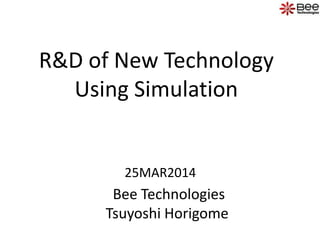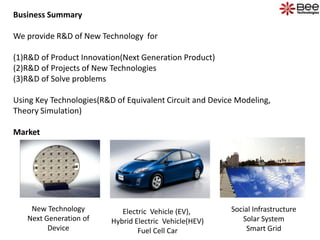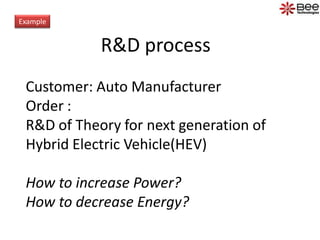Successfully reported this slideshow.

# R&D of New Technology Using Simulation

0

Share×
1 of 9
1 of 9

# R&D of New Technology Using Simulation

0

Share

R&D of New Technology Using Simulation by Bee Technologies

R&D of New Technology Using Simulation by Bee Technologies

## More Related Content

### Related Books

Free with a 14 day trial from Scribd

See all

### Related Audiobooks

Free with a 14 day trial from Scribd

See all

### R&D of New Technology Using Simulation

1. 1. R&D of New Technology Using Simulation Bee Technologies Tsuyoshi Horigome 25MAR2014
2. 2. Business Summary We provide R&D of New Technology for (1)R&D of Product Innovation(Next Generation Product) (2)R&D of Projects of New Technologies (3)R&D of Solve problems Using Key Technologies(R&D of Equivalent Circuit and Device Modeling, Theory Simulation) Market Social Infrastructure Solar System Smart Grid Electric Vehicle (EV), Hybrid Electric Vehicle(HEV) Fuel Cell Car New Technology Next Generation of Device
3. 3. R&D process Example Customer: Auto Manufacturer Order : R&D of Theory for next generation of Hybrid Electric Vehicle(HEV) How to increase Power? How to decrease Energy?
4. 4. R&D of Thinking Process STEP1 • Circuit Method Approach • Idea of Circuit Method STEP2 • Behavior of Device Method Approach • Idea of Equivalent Circuit of Semiconductor • Idea of Equivalent Circuit of Passive Parts STEP3 • Conclusion of Theory • Evaluate of Idea using Simulation • Report of R&D Inductor IGBT:55% Input Capacitor FWD
5. 5. Circuit Method Approach Thinking of Topology Thinking of Circuit Method using electrical and electronic Theory Thinking of Circuit Modeling Create Equivalent Circuit from Idea Evaluation of Equivalent Circuit using Mathematics level If Idea is OK, Next implement create Control Signal Method Evaluation of Equivalent Circuit using Circuit level Simulation Idea transfer Equivalent Circuit STEP1 R&D of Circuit Method Approach
6. 6. STEP1 R&D of Circuit Method Approach Topology TopologyOptimize Control Signal
7. 7. Behavior of Device Method Approach Thinking of Switching Device of Theory using Device Process Thinking of IGBT of Behavior Theory of Electric Characteristics Thinking of IGBT of Transfer Characteristics of Mathematics and create Equivalent Circuit Thinking of IGBT of Saturation Characteristics of Mathematics and create Equivalent Circuit Thinking of IGBT of Gate Charge Characteristics of Mathematics and create Equivalent Circuit Thinking of IGBT of Swithing Characteristics of Mathematics and create Equivalent Circuit Evaluation of Equivalent Circuit of IGBT using Mathematics level If Idea is OK, Equivalent Circuit using Circuit level Simulation Thinking of FWD of Forward IV Characteristics of Mathematics and create Equivalent Circuit Thinking of FWD of Reverse Recovery Characteristics of Mathematics and create Equivalent Circuit Thinking of –didt Method Evaluation of Equivalent Circuit of FWD using Mathematics level If Idea is OK, Equivalent Circuit of FWD using Circuit level Simulation Idea connect between IGBT and FWD Thinking of Switching loss of IGBT and FWD using Circuit Simulation Evaluation idea of theory of switching loss using Circuit Simulation If idea want to high level using Optimize Method for Power loss(Switching Loss) Next Step Thinking of idea for increase power(Energy concert) Thinking of Core and coil using Material Science , Electro Dynamics , Mathematics and Impedance analysis. Idea convert to Equivalent Circuit and evaluate idea using Circuit Simulation. Idea of energy conversion efficiency estimate calculation using simulation STEP2 R&D of Behavior of Device Method Approach
8. 8. STEP2 R&D of Behavior of Device Method Approach IGBT Input MOSFET M1 MFIN Q1 QOUT RE E DDS DO 81 DBE DE 85 RC 8.3m C RG G 82 CGE 83 83 VC 0Vdc IN+ IN- OUT+ OUT- E1 EVALUE C2 C1 0 R1 100MEG CGD 1 S1 MSW R2 100MEG D1 DGD S2 MSW 2 Idea of Equivalent Circuit IGBT Process Theory Idea of Function of IGBT using Mathematics Transfer Characteristics Output Characteristics ・ ・ ・ ・ ・ Gate Charge Characteristics
9. 9. STEP3 Conclusion Inductor IGBT:55% Input Capacitor FWD Simiulation Simiulation Simiulation Simiulation Condition: Vout=342V, ILoad=40A, Freq=10kHz - Power Loss Chart